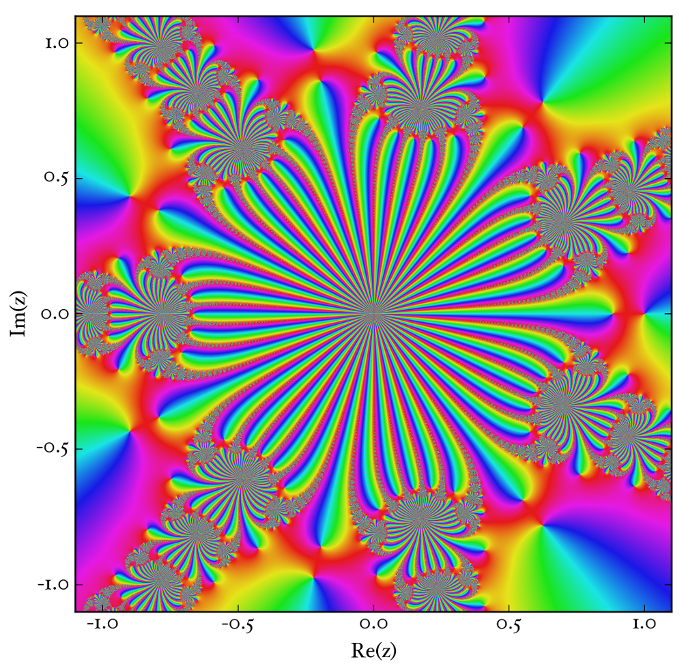Analytic Constant Microscopy

Say I have a constant, pi, or e, or what have you which: A. occurs in infinite series/products,  and B. infinite series/products representations for it exist

Ramanujan’s:

$latex \frac{1}{\pi} = \frac{2\sqrt{2}}{9801} \sum^{\infty}_{k=0} \frac{(4k)!(1103+26390k)}{(k!)^4 396^{4k}}$$\pi_{n} = \left[\frac{1}{\pi} = \frac{2\sqrt{2}}{9801} \sum^{n}_{k=0} \frac{(4k)!(1103+26390k)}{(k!)^4 396^{4k}}\right]^{-1}$

If the Dirichlet Eta function is

$latex \eta(\tau) = e^{\frac{\pi \rm{i} \tau}{12}} \prod_{n=1}^{\infty} (1-(e^{2\pi i \tau})^{n})$

Then a Ramanujan-pi-formula-approximant-deformed Dirichlet Eta function is:

$latex \eta(\tau) = e^{\frac{\pi \rm{i} \tau}{12}} \prod_{n=1}^{\infty} (1-(e^{2\pi_{n} i \tau})^{n})$

with$\pi_{n}$ as defined above.

Constructing new functions from root finding methods

Suppose I have a root finding method on some function$w(z)$ on the complex plane$\mathbb{C}$, okay, that gets us$\mathbb{C}$ partitioned into Wada basins, and more to the point, those basins are associated with particular roots of$w(z)$. Which means that if a basin is associated with the root$r$, then$w(r)=0$

Adding things that go to zero is a good way of making new functions. What if we were to take the sequence generated by the Newton’s  method iteration and then add together$w(z_{1}) +w(z_{2})+\ldots$? (or any other root-finding method.

for w(z) = 1-z^7, this gets us:If a root finding method is a rhizogeny, then I’m going to call the class of functions generated this way metarhizogenous.

Olfactory category/topos theory sensory articulations (warning: far out)

Let Ξ be some mathematical entity — be it topos or category or whatnot — with morphisms.

To each morphism of Ξ, assign a spring constant k (in practice, I’m hoping there are more or less canonical spring constants for each Ξ)

We could obtain a vibrational spectrum of Ξ (this is done in practice with small molecules, in which the vibrational spectrum is determined by the application of group theory) , and if Luca Turin’s theory of olfaction is correct

(http://en.wikipedia.org/wiki/Vibration_theory_of_olfaction), one could with the right

olfactory prosthesis (which is well beyond current technology) , smell the difference between FinSet, Grp, and nCob.

More to the point, the whole enterprise would no longer have the sensory qualia of a child in the 1950s playing with an erector set which contains morphisms and objects, and some flashlights (functors)

When you read about the olfactory sense of dogs, http://www.pbs.org/wgbh/nova/nature/dogs-sense-of-smell.html, I wonder just what exotic structures might exist that we could only get a whiff of if we could olfactorize Ξ and its congeners

fantasy machine

every once in a while I like to just sketch out future computing hardware. I recently got an nvidia 9500GT card. Soon I’ll likely try to get a GTX card (because those CUDA cores could rapidly accelerate my renders).

At least 32GB of RAM. Intel (at least an 8 core xeon, maybe more cores). Probably running raring.

4TB of spinning disk. 4TB of SSD storage.

The compute architecture of this hypothetical machine is going to be a little strange:

an nvidia tesla card of some sort

an nvidia gtx/quadro card for display

an amd firepro card

and an intel xeon phi card.

three of these cards are for computation purposes only. I can see how someone might balk at this, but hear out my reasons for this strangely architectured machine: individually, I’m not sure how much faith I want to put in a specific vendor. Yes, they’re all very fast, but my goal is not bitcoin mining or mmorpg playing, but doing mathematics visualizations. The heterogenous compute architecture in this hypothetical machine is insurance against hardware quirks by each vendor. One would like to verify computations on different hardware in the same way that it used to be the case that one would test supercomputers by instructing them to compute many pi to many a decimal place, because then the output could be tested against previous computations, and if there were mistakes, tracing them to problems of machine design or implementation was the thing to do.

tail equivalence in the category of series

Consider the category Series.

Say that two objects of Series are tail-equivalent if and only if their quotient with respect to their variables (as the index is increased without bound) is one.

For instance$\sum_{n=1}^{\infty} q^{n^{2}}$ and$\sum_{n=1}^{\infty} q^{n^{2}-q^{n}}$

We’ll write$\sum_{n=1}^{\infty} q^{n^{2}} \sim \sum_{n=1}^{\infty} q^{n^{2}-q^{n}}$

I believe$\sim$ is an equivalence relation. But lo, what of the quotient category Series/$\sim$